# Math Games by Domain

Grade 5 Math Games by Domain

*Click on the links to access Math Games*

Operations & Algebraic ThinkingWord Problems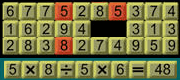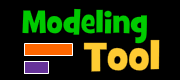Thinking BlocksExponents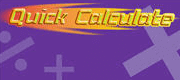Evaluate Expressions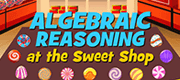Algebraic Reasoning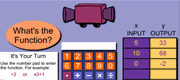Numerical Relationships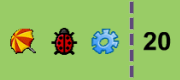Numerical PuzzlesCandy ChallengeCalculator Chaos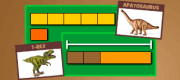Thinking Blocks

Numbers and Operations in Base 10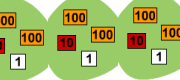Decimals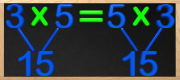Commutative Property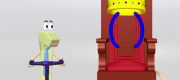Associative Property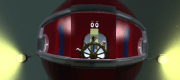Distributive Property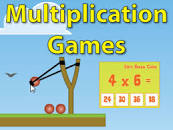Multiplying Two Digits by Two Digits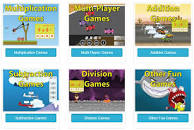Multiply 3 - 4 digits up to 100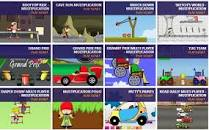Multiplication PropertiesDivision PatternsDivision with RemaindersDividing Greater Numbers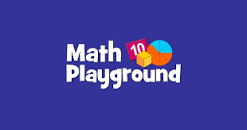Add and Subtract Decimals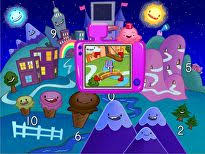Add and Subtract Decimals

Numbers and Operations with Fractions

Measurement & DataProbability and GraphingCube PerspectiveAlien Angles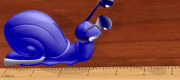Line PlotsAngles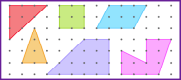GeoboardPie Chart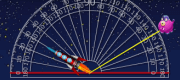Rocket Angles

Geometry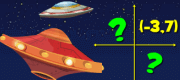Shape Names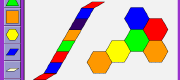Pattern BlocksRescue Mission